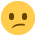# F.A.O Sandy

Hi Sandy,

I am attempting Dec 07 MAC Paper & am completely stuck on Task 1.1 F. It asks you to calculate the Budgeted OAR per machine hr and per game. I have looked at the answers but need an explanation as to how you would tackle a question like this. When you get a minute can you please explain it. Thanks.

P.S Where does the required machine hours figure of 1381.25 hrs come from in the answer?

This is confusing me big time!• Hope Sandy wouldn't mind.

I think you have missed this bit " each game requires the same time in production" then the total production budget for Ybox and siny games would be 152500+400000=552500,the number of games produced per machine hour is 400,then use the total production budget 552500 divided by 400 is 1381.25 machine hours required.

The fixed production overhead budget is 200,000*5+40,000*5=1200,000
the budgeted overhead absorption rate per machine hour is 1200,000/1381.25=868.78
the budgeted overhead absorption rate per game is 1200,000/552500=2.17
• Hope Sandy wouldn't mind.

I think you have missed this bit " each game requires the same time in production" then the total production budget for Ybox and siny games would be 152500+400000=552500,the number of games produced per machine hour is 400,then use the total production budget 552500 divided by 400 is 1381.25 machine hours required.

The fixed production overhead budget is 200,000*5+40,000*5=1200,000
the budgeted overhead absorption rate per machine hour is 1200,000/1381.25=868.78
the budgeted overhead absorption rate per game is 1200,000/552500=2.17

Thanks for that now to study it and see if it clicks! lol